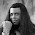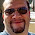## Friday, December 4, 2015

### HP Prime: Gauss-Jordan Elimination Method

HP Prime:  Gauss-Jordan Elimination Method

I received an email requesting some programs of various numerical methods.   One of the methods is the Gauss-Jordan Elimination Method.

Basically, the Gauss-Jordan Elimination Method is a step-by-step method of matrix row operations to reduce a matrix A = [ X | Y ] where X is (mostly) a square component joined by a column vector Y to the form [ I | R ], which I represents an identity matrix portion where the diagonal elements are 1.

You can quickly execute the method by use of the RREF (Reduced Row Echelon Form) function, which is present on many graphing calculators (if not all of them these days).

The HP Prime program GAUSSJORDAN is shows a step by step method.  What the program does is:

1. Take the dimensions of the matrix.
2. Starting with column 1, pivot on element (1,1).  This is accomplished by one of two operations.  SCALEADD which multiplies the first row by a factor and adds it to a target row k.  The goal is reduce the element (k,1) to 0, for all k ≠ 1.
3. Once step 2 is completed, the SCALE command is used to divide the value of element (1,1) to reduce it to 1, if necessary.
4. Repeat steps 2 and 3 for each column until the number the rows is reached.

Notes:

1. HP Prime’s SCALEADD is the *Row+ command for the Casio and TI graphing calculators.  Similarly, HP Prime’s SCALE is the *Row command for the Casio and TI graphing calculators.
2. The program requires that the matrix have all elements that are (1,1), (2,2), (3,3), etc. are non-zero.  Otherwise an error occurs.  You can swap rows by the rowSwap command if necessary to get the matrix in proper form.
3. While the program GAUSSJORDAN shows you step by step, it shows one order of approach, which may or may not be the most efficient number of steps.
4.  If the HP Prime is in Standard mode, you may see 0.9999999999999 or some number to the 10^-13 power.   This is due to the rounding mechanisms.  Feel free to round these numbers to 1 and 0, respectively.

With all this in mind, here is the program:

Program GAUSSJORDAN

EXPORT GAUSSJORDAN(mat)
BEGIN
// Guass-Jordan Elimination
// 2015-12-04
// Matrix MUST have
// mat[k,k]≠0

LOCAL l,r,c,j,k,h,v;
PRINT();

l:=SIZE(mat);
r:=l; c:=l;
j:=1;

// Main row loop
FOR k FROM 1 TO r DO

// Secondary row loop
FOR h FROM 1 TO r DO

// k = target row
// h = test row
// Pivot operation
IF h≠k THEN
v:=−mat[h,k]/mat[k,k];
PRINT();
PRINT("After Step "+j);
PRINT(mat);
WAIT(0);
j:=j+1;
END;

IF mat[k,k]≠1 THEN
v:=1/mat[k,k];
mat:=SCALE(mat,v,k);
PRINT();
PRINT("After Step "+j);
PRINT(mat);
WAIT(0);
j:=j+1;
END;

END;
END;

PRINT();
PRINT("Result:");
PRINT(mat);

// Final WAIT not needed

RETURN mat;

END;

Examples:

M2 = [[1,2,4,1] [3, -3, 3, 0] [6, 6, 8, 5]]
Final Result* (see Note 4) of GAUSSJORDAN(M2):
[[1,0,0,0.53333333333]. [0,1,0,0.43333333333], [0,0,1,-0.1]]

M3 = [[0,4,-1,-1], [2,5,2,2], [3,3,6,3]].
Running GAUSSJORDAN(M3) with M3 as is will get an error.  Why?  See M3[1,1] = 0.  We must make it non-zero.  Do this by swapping rows.
M3≔rowSwap(M3,1,2) to make the matrix [[2,5,2,2], [0,4,-1,-1], [3,3,6,3]].  Now you are ready to go.
GAUSSJORDAN(M3) returns [[1,0,0,2.6], [0,1,0,-0.4], [0,0,1,-0.6]]

Thank you Luis for the email and the suggestions.

Source:  Cazelais, Gilles. “Gauss-Jordan Elimination Method”  http://pages.pacificcoast.net/~cazelais/251/gauss-jordan.pdf  Retrieved December 4, 2015

Until next time, stay safe everyone because it is a crazy planet we live on.

Eddie

1.Hi Edward,
no more programs for HP 15 or 11 ?

1.I wouldn't say no more programs for the HP 15/11. But thank you for reminding me because those calculators (15 in particular since I don't own an 11) have been put on the back burner.

2.Hello
I am looking for a way to program the HP prime to return 2 or more roots of an equation. Example in my program i use Solve.SOLVE (D=A*X,X) and it returns the value of X. I want to do the same thing in my program but for A=D*T+X^3 and return 3 values which i can use later on in my program.
Thank you

3.Thank you for sharing information about optima scales . I would be thankful if you share more information about prime scales .
prime scales

### Retro Review: Radio Shack EC-2024

Retro Review:  Radio Shack EC-2024 Quick Facts Company:  Radio Shack Years in Production: Around 1994 Original Price: \$24.99 Bat...